# Calculate the voltage between points A and B

## Homework Statement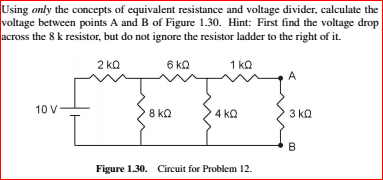## Homework Equations

/ 3. The Attempt at a Solution [/B]
Here is my attempt at the homework, I'm trying to correct my mistakes and am looking for help in the right direction.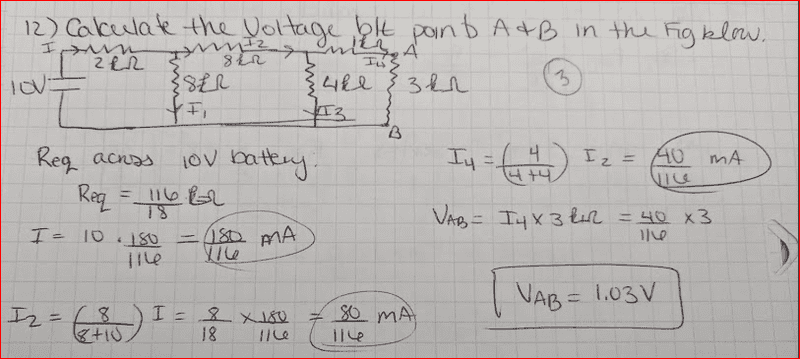cnh1995
Homework Helper
Gold Member
Use the given hint. You need not find the current at all. Simplify the network and find the voltage across the 8 ohm resistor first, using voltage divider.

CWatters
Homework Helper
Gold Member
+1 to using the hint.

The values of the resistors were carefully selected by the problem setter to make simplifying the network easy.

Can you reduce it this far?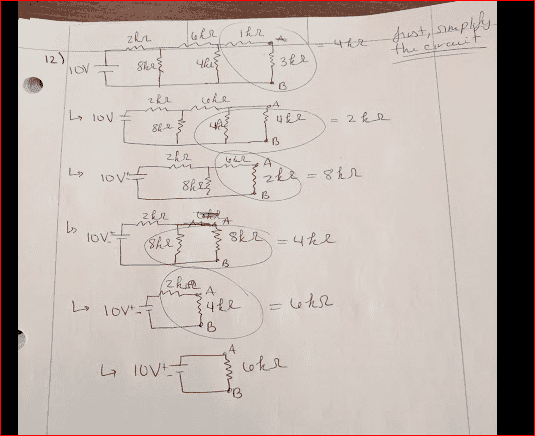gneill
Mentor
All good until that last operation: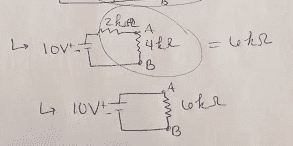The point A on the last circuit is not the point A from the circuit before. By combining the final two resistors you've "lost" point A. What you've done is reduce the entire load to a single resistance. While this would allow you to find the total current supplied by the 10 V source, it won't let you find the voltage AB.

Looking at the first circuit in the figure, what technique might you apply to find the voltage AB?

Gotcha, I questioned if that were allowed!
I know from Ohm's law that V=IR so Vab= I*R
And if we know that we start with a rise of 10V until the 2kOhm resisitor, could we find the current, voltage at R1 then use that to find the Voltage at R2?

gneill
Mentor
Gotcha, I questioned if that were allowed!
I know from Ohm's law that V=IR so Vab= I*R
And if we know that we start with a rise of 10V until the 2kOhm resisitor, could we find the current, voltage at R1 then use that to find the Voltage at R2?
In other words, apply the voltage divider concept? Sure! It's one of the concepts that the problem statements says you're to use.

OK!
So would this be correct then?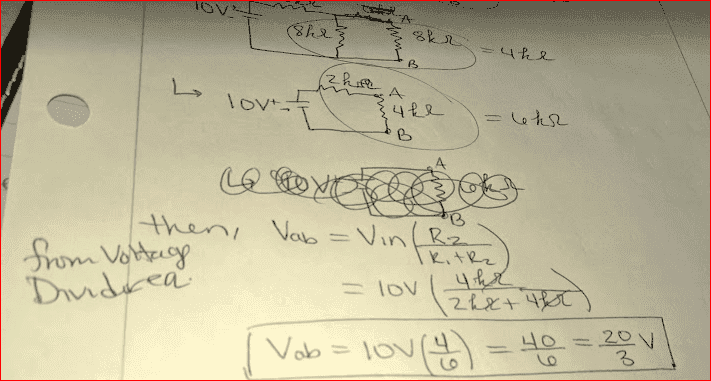Node A kind of disappeared when you added the 1k and 3k resistors in series. So the voltage you found (20/3 V) is the voltage at the node that the right hand side of the 2k resistor connects to. But that is not node A. But you have made progress. You calculated the voltage at that point.

So, can you not reduce the circuit at all in this example without losing points A or B?
Or is it allowable to reduce from the left side?
e.g. that the 2kOhm and 8kOhm resistors become a 10kOhm resistor, and the 6kOhm and 4kOhm resistor become another 10kOhm resistor?

So, can you not reduce the circuit at all in this example without losing points A or B?
When you combine resistors in series, you lose a node. But that's okay. Now you have more information than when you started. You know that the voltage at the right-hand side of the 2k resistor is 6.667 V. So just continue from there until you are able to figure out the voltage at node A.

Or is it allowable to reduce from the left side?
e.g. that the 2kOhm and 8kOhm resistors become a 10kOhm resistor, and the 6kOhm and 4kOhm resistor become another 10kOhm resistor?
I think I was editing this just as you were posting your response :)
Would this be allowed?

No. The 2k and 8k are not in series because there is something else that is connected in between them. You are on the right track. Just repeat what you did to find the 6.667 V. Simplify the circuit again until you can calculate another point. Edit: I should have said "node" instead of point.

I recommend that you start from the right-hand side again and do exactly what you did before. But stop reducing when you have a voltage divider that you can calculate that will give you the voltage at the next node to the right of the 6.667 V node that you just calculated.

Can I even reduce to it from the right, then? I keep thinking that will just eliminate node A again?

I sometimes find it easier to re-sketch the circuit as branches, with all the elements facing one direction: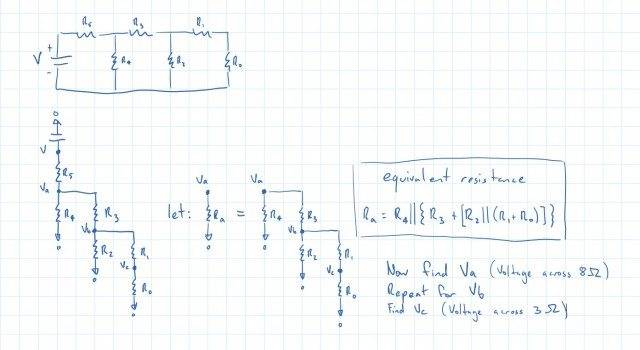gneill
Mentor
Can I even reduce to it from the right, then? I keep thinking that will just eliminate node A again?
The idea is that you are reducing the circuit in stages from the left until you are left with a just a voltage divider that contains the original nodes A and B. It may not be obvious that this is the case until after you've done the first iteration.

You started by finding the equivalent resistance that parallels the 8 kΩ resistor, reducing that pair to a single resistance and applying the voltage divider rule to the reduced circuit: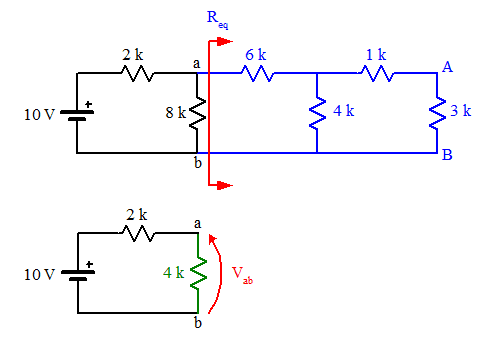Note that the nodes a and b are not A and B. What you've found is the voltage Vab that will appear across the 8 kΩ resistor in the original circuit. With that you can replace everything to the left of the red dividing arrows with a voltage source equal to Vab: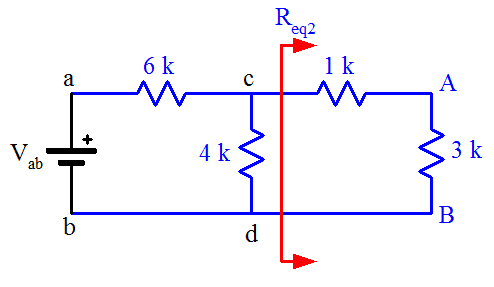and you're ready to repeat the process to find the voltage Vcd.

•CWatters